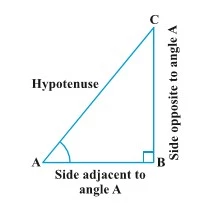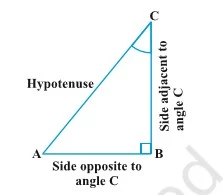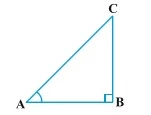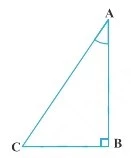Click Question to Get Free Answers >

# Introduction To Trigonometry NCERT Solutions : Class 10 Maths

## NCERT Solutions for Class 10 Maths : Introduction To TrigonometryFilter
Filters :
Classes
•
•
•
•
•
•
•
Chapters
•
•
•
•
•
•
•
•
•
•
• 5 More
Exercises
•
•
•
•
•

### NCERT Class 10 | INTRODUCTION TO TRIGONOMETRY | Exercise 02 | Question No. 01

Evaluate the following (i) `sin 60^@ cos30^@ + sin 30^@ cos 60^@` (ii) `2tan^2 45^@ + cos^2 30^@ - sin^2 60^@` (iii) `(cos45^@)/(sec30^@ + c o s e c 30^@)` (iv) `(sin30^@ + tan45^@ - c o s e c 60^@ )/(sec30^@ + cos60^@ +cot45^@)` (v) `(5cos^2 60^@ + 4sec^2 30^@ - tan^2 45^@ )/ (sin^2 30^@ + cos^2 30^@ ) `Doubtnut one of the best online education platform provides free NCERT Solutions of Maths for Class 10 Introduction To Trigonometry which are solved by our maths experts as per the NCERT (CBSE) guidelines. We provide the solutions as video solutions in which concepts are also explained along with answer which will help students while learning , preparing for exams and in doing homework. These Solutions will help to revise complete syllabus and score more marks in examinations.

Get here free, accurate and comprehensive NCERT Solutions for Class 10 Maths Introduction To Trigonometry which have been reviewed by our maths counsellors as per the latest edition following up the CBSE guidelines. We provide video solutions in which solutions to all the questions of NCERT Class 10 Maths textbook are explained in step by step and detailed way.

## The Topics of the Chapter Introduction To Trigonometry are :

TRIGONOMETRIC RATIOS OF SOME SPECIFIC ANGLES

TRIGONOMETRIC IDENTITIES

TRIGONOMETRIC RATIOS

TRIGONOMETRIC RATIOS OF COMPLEMENTARY ANGLES

SOME COMPLEX QUESTIONS

INTRODUCTION

It contains these exercises along with solved examples. All the exercises are solved in the video. Select the exercise to view the solutions exercisewise of the Chapter Introduction To Trigonometry:

## NCERT Solutions Class 10 Maths Chapter Introduction To Trigonometry Exercises:

We have covered all the exercises and also Solved examples in the videos. Along with the practise exercise Students should also practise solved examples to clear the concepts of the Introduction To Trigonometry. If incase you have any doubt you can watch the solutions for the given questions.Watch the solutions for the given questions in which it is also explained in the video steps to solve the questions along with answers.

In this chapter, you will learn about trigonometric ratios, trigonometric ratios of specific angles, trigonometric ratios of complementary angles, etc. Trigonometry can be stated as the calculation section of geometry. In trigonometry, you apply knowledge of triangles from geometry and use the formulas to help you solve problems. It is quite important for the students to get the right and clear idea of the different terms that prove to be quite useful in solving the problems. Doubtnut has made it more interesting with examples and solved exercises. This helps you to get rid of your queries in the best manner.

CBSE curriculum utilizes NCERT books for imparting quality education to the students However, NCERT books help students to clear their basic concepts on different terms or topics. But it lacks the right type of information which is required by you to attain the comprehensive idea of the particular concept. So, for this, attempting the tricky questions set in the examinations proves to be quite confusing by the students. In order to achieve good marks in the subject, they try to get reference books. By doing so, they manage to get some good benefits out of it.

Considering the problems which are faced by the you in getting your queries cleared, a team of expert tutors of Doubtnut has made it possible to provide an easy learning method for you. They have introduced online study materials that you can access from anywhere else. With the best step by step instructions by the experts, students get hold of all the right and accurate details to clear their queries. Learning has become fun and easy where it also leads to building up a good confidence level in the right manner. So, students willing to score better marks in their subject should definitely try to opt for Doubtnut’s video tutorials for sure without fail. They can also get access to the most useful Class 10 Maths NCERT Solutions that proves to be an added advantage to them.

## Topics and subtopics included in NCERT Solutions for Class 10 Maths Chapter 8 Introduction to trigonometry

### Introduction

In your earlier classes, you have studied about triangles including right angles. Here, we will focus on trigonometric ratios of the angle. However, we will try to restrict our discussion to acute angles only.  We will also calculate trigonometric ratios for some specific angles as well.

### Trigonometric ratios

Let us have a look at the right triangle ABC.Here, ∠ CAB (or, in brief, angle A) is an acute angle. Note the position of the side BC with respect to angle A. It faces ∠ A. We call it the side opposite to angle A. AC is the hypotenuse of the right triangle and the side AB is a part of ∠ A. So, we call it the side adjacent to angle A.Note the position of sides change when you consider angle C in place of A in the above figure.

You have been taught the concept of ‘ratio’ in your earlier classes. Below are some certain ratios involving the sides of a right triangle, and we would call them trigonometric ratios.

The trigonometric ratios of angle A in the right triangle ABC are defined as follows :

sine of ∠ A =  side opposite to angle A = BC

hypotenuse                AC

cosine of ∠ A = side adjacent to angle A = AB

hypotenuse                  AC

tangent of ∠ A =        side opposite to angle A = BC

side adjacent to angle A   AB

cosecant of ∠ A  = _ 1         =          hypotenuse             = AC

Sine of ∠ A    side opposite to angle A      BC

secant of ∠ A = _ 1                =         hypotenuse             = AC

Cosine of ∠ A    side adjacent to angle A       AB

cotangent of ∠ A= _    1            = side adjacent to angle A = AB

tangent of ∠ A     side opposite to angle A  BC

The aforementioned ratios are abbreviated as sin A, cos A, tan A, cosec A, sec A and cot A respectively. Note that the ratios cosec A, sec A, and cot A are respectively, the reciprocals of the ratios sin A, cos A, and tan A.

BC

Also observe that tan A = BC = AC = sin A and cot A = cos A

AB     AB    cos A                   sin A

AC

So, the trigonometric ratios of an acute angle in a right triangle defines the relationship between the angle and the length of its sides.

### Trigonometric ratios of Specific Angles

You are familiar with the construction of angles of 450, 600, 900, etc. taught  from geometry unit. Here we will get to know how to find the values of the trigonometric ratios for the different angles. Let’s find out the trigonometric ratios of 450In âˆ† ABC, right-angled at B, if one angle is 45°, then the other angle is also 45°, i.e., ∠ A = ∠ C = 45°

So, BC = AB

Now, Suppose BC = AB = a.

Then by Pythagoras Theorem, AC2 = AB2 + BC2 = a2 + a2 = 2a2,

and, therefore, AC = Square root of 2 a ⋅

Using the definitions of the trigonometric ratios, we have :

sin 450side opposite to angle 450  = BC =        a               =        1      .

hypotenuse              AC   a square root 2      square root2

cos 450 = side adjacent to angle 450 = AB =          a             =      1       .

hypotenuse              AC    a square root 2   square root 2

tan 450side opposite to angle 450 = BC = a = 1

side adjacent to angle 450    AB    a

cosec 450      1      = square root 2

sin 450

sec 450         1        = square root 2

cos 450

cot 450   =       1   = 1

tan 450

### Trigonometric ratios of Complementary Angles

As we know that two angles are said to be complementary if their sum equals 900In âˆ† ABC, right-angled at B, are there any complementary angles?

Since ∠ A + ∠ C = 90°, they form such a pair. We have:

sin A = BC                                cos A = AB

AC                                            AC

tan A = BC                           cosec A = AC

AB                                           BC

---------------------- (1)

sec A = AC                             cot A =  AB

AB                                          BC

The trigonometric ratios for ∠ C = 90° – ∠ A.

Here we shall write 90° – A instead of 90° – ∠ A.

What would be the side opposite and the side adjacent to the angle 90° – A?

You will find that AB is the side opposite and BC is the side adjacent to the angle 90° – A. Therefore,

sin ( 900- A) = AB,                 cos(900- A) = BC

AC                                       AC

tab ( 900- A) = AB,                 cosec ( 900- A) = AC

BC                                           AB                                                                                                                                                                ...........(2)

sec ( 900- A) = AC                     cot ( 900- A) = BC

BC                                           AB

Now, compare the ratios in (1) and (2). Observe that:

sin ( 900- A) = AB = cos A    and cos ( 900- A) = BC = sin A

AC                                              AC

Also tan (900- A) = AB = cot A     cot ( 900- A) = BC = tan A

BC                                      AB

sin ( 900- A) = AC = cosec A    and cosec ( 900- A) = AC = sec A

BC                                                    AB

So, sin (90° – A) = cos A,                     cos (90° – A) = sin A,

tan (90° – A) = cot A,                           cot (90° – A) = tan A,

sec (90° – A) = cosec A,                        cosec (90° – A) = sec A,

for all values of angle A lying between 0° and 90°. Check whether this holds for A = 0° or A = 90°.

Note : tan 0° = 0 = cot 90°, sec 0° = 1 = cosec 90° and sec 90°, cosec 0°, tan 90° and cot 0° are not defined.

### Trigonometric identities

Here we will prove one trigonometric identity that would also prove other useful trigonometric identities.In âˆ† ABC, right-angled at B, we have: AB2 + BC2 = AC2                       (1)

Dividing each term of (1) by AC2, we get

AB2 +BC2 = AC2

AC2    AC2     AC2

i.e., (cos A)2 + (sin A)2 = 1

i.e., cos2 A + sin2 A = 1                                                           (2)

This is true for all A such that 0° ≤ A ≤ 90°. So, this is a trigonometric identity.

Let us now divide (1) by AB2. We get:

AB2 +BC2 = AC2

AB2    AB2     AB2

i.e., 1 + tan2 A = sec2 A                                                           (3)

Is this equation true for A = 0°? Yes, it is. What about A = 90°? Well, tan A and sec A are not defined for A = 90°. So, (3) is true for all A such that 0° ≤ A < 90°.

Let us see what we get on dividing (1) by BC2. We get:

AB2 +BC2 = AC2

BC2    BC2     BC2

i.e., cot2 A + 1 = cosec2 A                                                        (4)

Note that cosec A and cot A is not defined for A = 0°. Therefore (4) is true for all A such that 0° < A ≤ 90°.

With the use of these identities, each trigonometric ratio can be expressed in terms of other trigonometric ratios, i.e., if any one of the ratios is known, we can also determine the values of other trigonometric ratios.

## Significance of Doubtnut for Class 10 students

By introducing the most helpful video tutorials by the team of Doubtnut, it has made the students get all the perfect idea on how to attempt the different questions. All efforts have been made to provide the best NCERT Class 10 Maths Solutions Chapter 8. Well, there are also other advantages that students can enjoy by looking forward to the best and useful online study materials.

• Enjoy flexibility: The study materials prepared by the experts of Doubtnut prove to be quite flexible for the students. The study materials can be downloaded so that one can try to access it as per their convenience. In other words, it is possible to go through the lessons no matter where they are. Thus, it proves to be quite flexible which does not lead to any problem at all.
• Provides the ultimate support: Getting hold of the best support is also possible where it helps the students to get the right idea on how to come up with the perfect solutions. This helps them a lot to stay confident on how to work on different questions. This also does not take much time to attempt even the most difficult questions that are set in the examination.
• Get Q & A practice: Students can also get lots of examples and that too with solved exercises enabling them in solving the complex questions. As math requires a lot of practice, the study materials provided by the experts truly help a lot to get the ultimate idea about the mathematical concept. It helps to score good marks in the examinations.